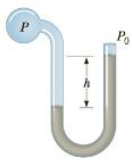Chapter 9, Problem 15P

Chapter
Section
Textbook Problem

A sphygmomanometer is a device used to measure blood pressure, typically consisting of an inflatable cuff and a manometer used to measure air pressure in the cuff. In a mercury sphygmomanometer, blood pressure is related to the difference in heights between two columns of mercury.The mercury sphygmomanometer shown in Figure P9.15 contains air at the cuff pressure P. The difference in mercury heights between the left tube and the right tube is h = 115 mmHg = 0.115 m, a normal systolic reading. What is the gauge systolic blood pressure Pgauge in pascals? The density of mercury is p = 13.6 × 103 kg/m3 and the ambient pressure is P0 = 1.01 × 105 Pa.Figure P9.15

To determine
The gauge systolic blood pressure Pgauge in Pascal.

Explanation

Given info: The density of mercury is 13.6×103kg/m3 , acceleration due to gravity is 9.80m/s2 , and the difference in mercury heights between the left and right tubes is 0.115m .

Explanation: The gauge pressure is defined as Pgauge=PP0=ρgh .

The formula for the gauge systolic blood pressure is,

Pgauge=ρgh

• ρ is density of mercury.
• g is acceleration due to gravity.
• h is the difference in mercury heights between the left and right tubes.

Substitute 13.6×103kg/m3 for ρ , 9.80m/s2 for g , and 0

Still sussing out bartleby?

Check out a sample textbook solution.

See a sample solution

The Solution to Your Study Problems

Bartleby provides explanations to thousands of textbook problems written by our experts, many with advanced degrees!

Get Started